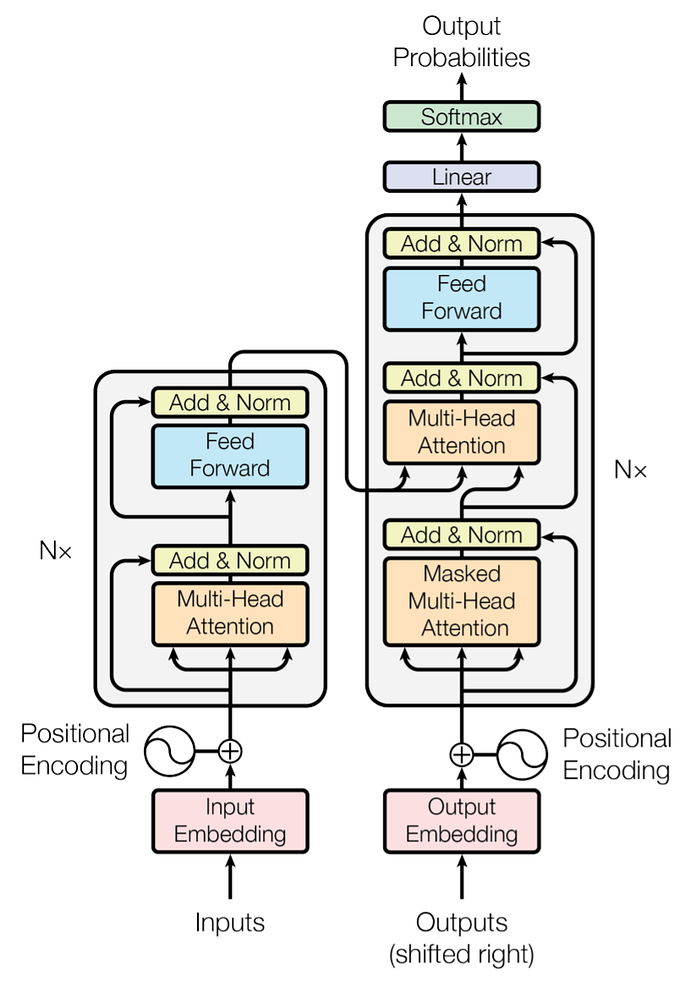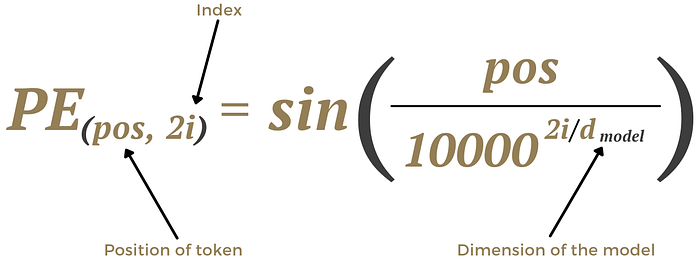• ## Study finds diversity in data science teams is key in reducing algorithmic bias

And no, not that type of Transformer.

# Background Information on Artificial Intelligence

Artificial Intelligence, as the name suggests, is imitating human intelligence. This can take the form of machine learning, but also if-then rules and decision trees. To continue, Machine Learning is a subset of A.I. that can be defined as an algorithm having the ability to learn without being explicitly programmed. Furthermore, Deep Learning is a subset of machine learning where the program can train itself.

Deep learning relies on multilayered artificial neural networks (ANNs). These neural networks are composed of varying neurons analogous to human neurons.

There are assorted types of ANNs, including convolutional neural networks (CNNs), multiplayer perception (MLP), and recurrent neural networks (RNNs).

The process of giving machines the ability to learn and think is increasingly becoming more and more streamlined due to advances made in neural networks.

This is a “mostly complete” chart of the different neural network architectures. A little overwhelming, I know.

A Transformer is a type of deep learning model. To preface, there are many neural networks out there, but what makes transformers notable is their attention modules and parallelization abilities.

“What are those!?!?” you may be asking. Well, I will elaborate on this later on. But first…

# The Problem

Recurrent Neural Networks or RNNs are a type of neural network used predominantly for natural language processing and speech recognition. On top of that, there are Long/Short Term Memory (LSTM) and Gated Recurrent Units (GRU) used in similar applications.

The issue with these ANNs is:

• The Speed of Training,
• How Accurate the Model is, and
• The Lengths of Data it can handle.

# The Solution

A paper called “Attention is All You Need,” published in 2017, introduces a different architecture.

This entire architecture was coined the Transformer and addresses these issues through their different components.Picture of Transformer Architecture || Courtesy of Attention Is All You Need

The Transformer comprises an encoder block (left) and a decoder block (right). The popular transformer model GPT-2 uses only the decoder portion, while BERT uses just the encoder block, accomplishing different NLP tasks.

# Overview of How the Encoder Block Works

Let’s use translating from English to French as an example to break down further what this picture means.

Computers don’t understand words or phrases, something comparatively simple as “I am cold.” To utilize this input, it’s tokenized and put through Input Embedding.

This is where every word is mapped to a point in space called the embedding space. Similar words with similar meanings are physically closer. In the embedding space, tokens are converted to vectors based on where it is.

In a Transformer, all of these inputs are put into the architecture simultaneously, causing it to lose its positional value.

A sentence such as: Only he told his wife that he loved her.” and “He told his wife that only he loved her.” both contain the exact words but have different meanings based on their position.

This is where the positional encoding comes in. We add positional encodings to each word embeddings.

Positional encoders are a vector that contains context on the relative position of the token/word.

In the original paper, the Positional Encoder uses both sine and cos functions.The same applies with cos function (swap sin out for cos)

The logic behind this is that the positional encoder formula must be based purely on position, can’t output repeated values, and be ≤ 1.

This matrix containing context is fed into the Encoder Block which consists of:

• Feed-Forward Layer (FNNs)

The Multi-Head Attention Layer generates attention vectors based on the question: What part of the input should it focus on?

The Position-wise Feed-Forward layer is a fully connected feed-forward network applied to each attention vector one at a time.

The Feed-Forward Network applies two linear transformations with a ReLU activation in between. These linear transformations are the same across different positions, making it “Position-wise” but use other parameters from layer to layer.

The transformation is applied one at a time. Since the attention nets are independent, parallelization can be used as demonstrated below.

How does this whole concept of attention work?

How do our embedding matrices become matrices with “attention”?

Multi-Head Attention consists of several Scaled Dot Product Attention layers operating in unison.

The process of Scaled Dot Product Attention can be broken up into many steps:

1. Query, Key, and Value matrices are created

This is done using the input vectors (input embeddings in the Encoder) to calculate the attention vector. These matrices each represent different abstractions or features of an input.

Embeddings are packed into matrix X to increase efficiency

Then the embeddings are multiplied with three weight matrices trained during the training process.

2. Calculating the score

The score is the dot product of the current query matrix and all the key matrices.

3. Divide the scores by the square root of the dimension of key vectors

4. Softmax

The result is passed through a softmax operation which turns the results from the scores into possibilities.

5. Multiply the value vectors by Softmax Output

These past few steps can be condensed into the equation:

The last step is summing up the weighted value vectors. This result is the output of the self-attention layer.

Multiple sets of weight matrices create multiple attention vectors for Multi-Headed Attention. Each set of weight matrices creates a different set of Q, K, and V vectors.

Another weighted matrix is used on the attention vectors to create one output for the feed-forward layer.

This also takes averaged vectors of each token in relation to other tokens because we want a quantified vector of the interactions. Instead, if it were just vectors, it would place too much emphasis on its relation with itself.

# Putting it Together: Encoder-Decoder Transformer

The Decoder Block is incredibly similar to the Encoder Block with an additional component.

It’s comprised of:

• the Multi-Head Attention Layer, and
• Feed-Forward Layer

K and V values derived from the Encoder’s output are fed into the Decoder’s second attention layer as the Key and Value matrices needed for Attention. The Q values come from the Decoder’s Masked Attention Layer.

These inputs are the past outputs from the Decoder block. With our example of English to French Translation, these are French words.

French words are put through the Output Embedding, going through a similar process as the input embedding to generate meanings that the computer can recognize. This also has a positional encoding creating context.

The first input into the Decoder block is a unique start token used to generate the first output. The final output generates a particular end token signifying the end of a sentence in our translation example.

After calculating the score, the masked inputs are created by applying an attention matrix. This matrix has negative infinity values when used; change the values of the tokens also to -inf. After the softmax layer, the -inf values turn to zero.

Turning the values into zeros causes the following block to be unable to use them.

After every layer, there’s addition and normalization applied.

The addition portion creates a residual connection to the input. This is done by taking the input (values before the specific layer) and adding it to the output.

# Normalization

The most commonly used normalization is typically batch normalization.

Batch normalization averages (as the name suggests) for each batch. The mean and the variance is calculated for each mini-batch.

Transformers (and many RNN models) use Layer Normalization instead.

Layer Normalization averages each layer, therefore not needing to rely on mini-batches.